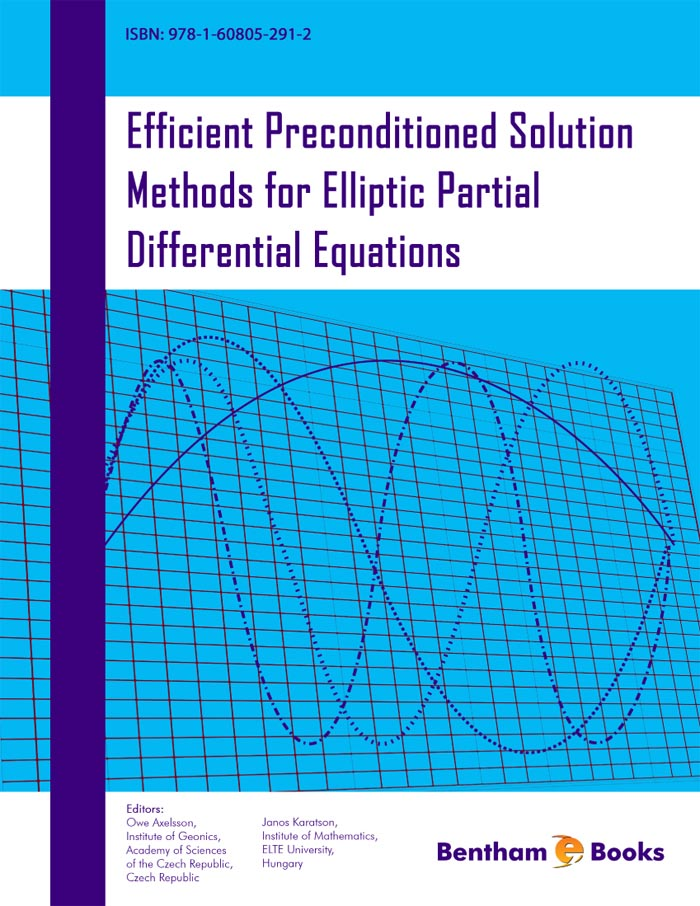### Construction of Preconditioners by Mapping Properties for Systems of Partial Differential Equations

#### Abstract

&lt;p&gt;The purpose of this chapter is to discuss a general approach to the construction of preconditioners for the linear systems of algebraic equations arising from discretizations of systems of partial differential equations. The discussion here is closely tied to our earlier paper , where we gave a comprehensive review of a mathematical theory for constructing preconditioners based on the mapping properties of the coefficient operator of the underlying differential systems. In the presentation given below we focus more on specific examples, while just an outline of the general theory is given.&lt;/p&gt;

Total Pages: 66-83 (18)